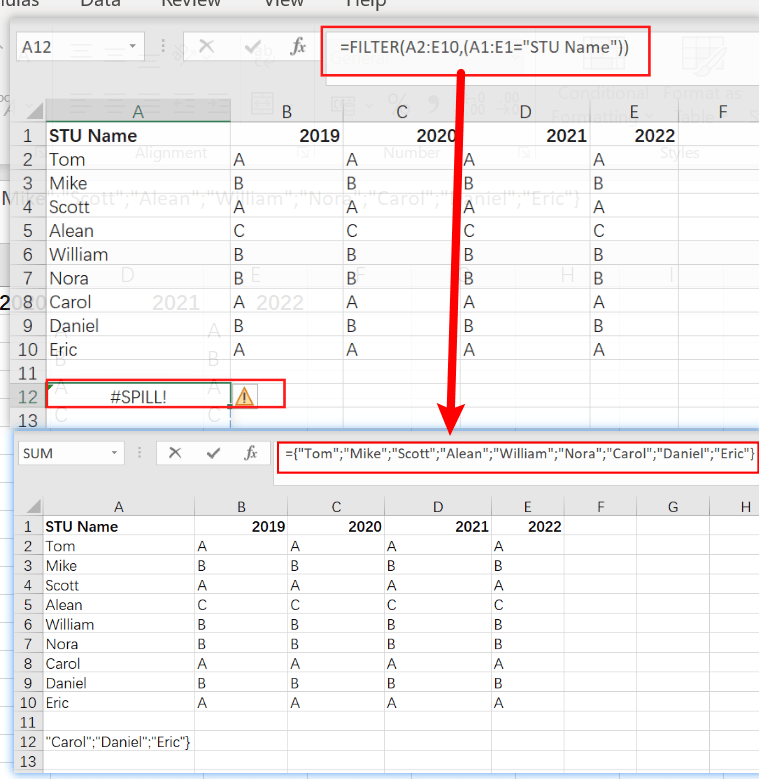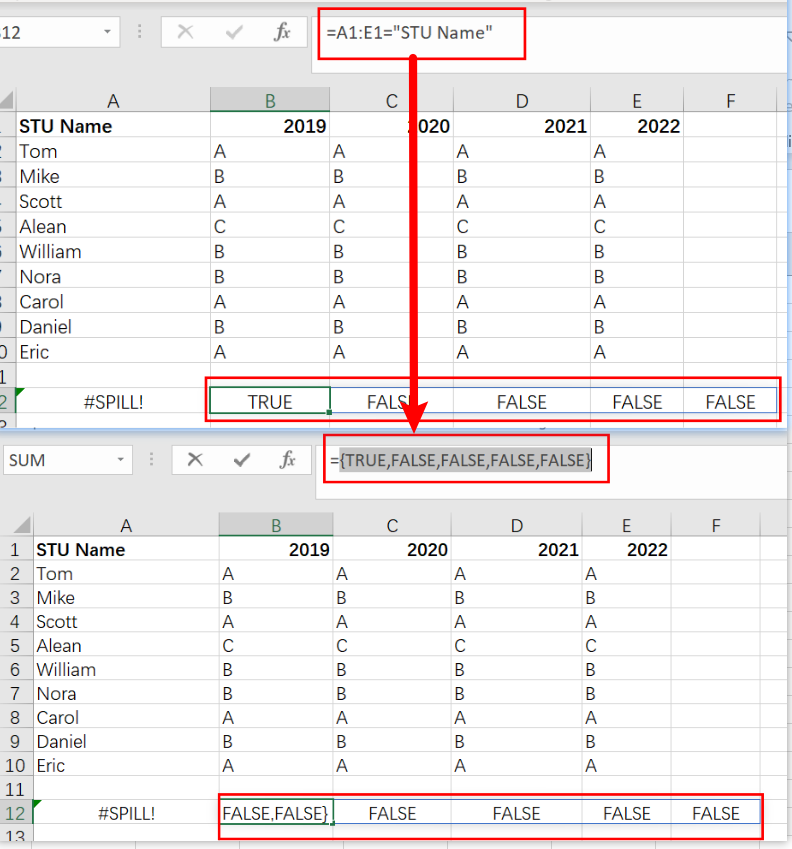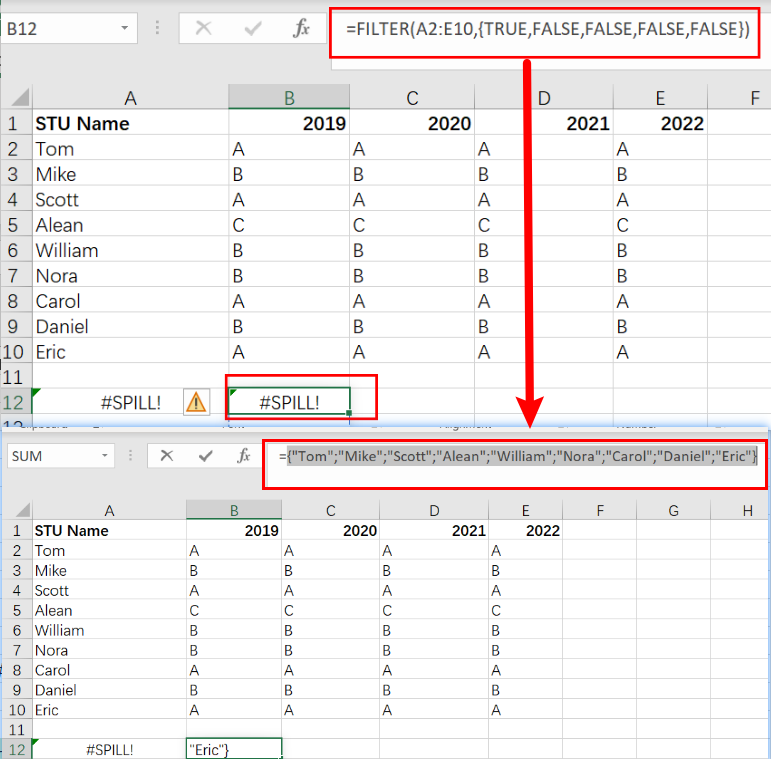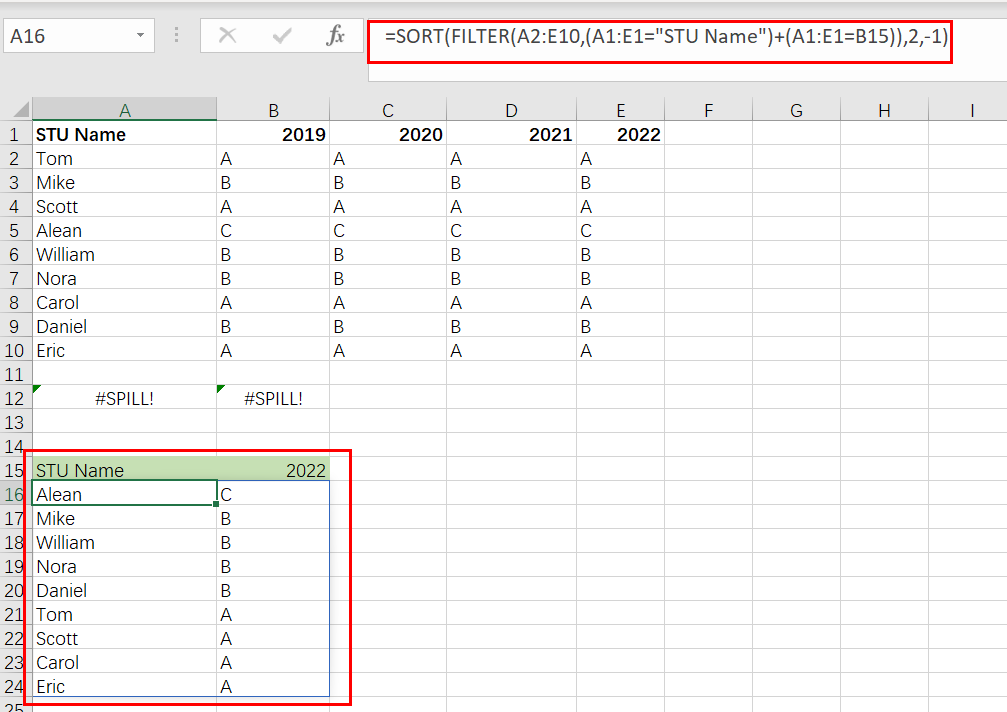# Filter Data by Column and Sort by Row

This post will guide you how to use the FILTER function to filter a value by column and then sort data by row in Microsoft Excel. You can use the following formula based on the SORT function in combination with the FILTER function to filter data by column and the sort data by row in Excel. the below is the general formula:

`=SORT(FILTER(Range,(Table_Header_Range= oneHeader)+(Table_Header=oneHeader)),2,-1)`

Or

`=SORT(FILTER(A2:E10,(A1:E1="STU Name")+(A1:E1=B15)),2,-1)`

## Let’s See How This Formula Works:

With this formula, you can sort and filter by column.

1) The heading `(A1:E1="STU Name")+(A1:E1=B15)` will keep the `STU Name` headings and add a `year` as a column heading, so there is a list of years for each `STU Name`.

2) `-1` means to sort from smallest to largest. If you want to arrange value from largest to smallest, use `1` instead.

3) In the end, it will take all your data that has a value in that year and show it from smallest to largest. It will filter out all the others since they do not have a value inside them for that row/column combination, hence the term Filter By Column Formula!

In the below example, the formula in A16 is:

`=SORT(FILTER(A2:E10,(A1:E1="STU Name")+(A1:E1=B15)),2,-1)`

The above formula returns the `STU Name` column plus data for a year in B15, sorted by values.

Note: The FILTER function in Excel 365 is a new and improved way to restrict data. In earlier versions of the program, some alternatives could be used, but they were more complex than what you’ll find with this handy addition!

We’ll filter the data shown in `A2:E10` by year and then sort it in this example. We also need to make sure that `STU Name` is included as well sorted in descending order like all of our other results! It is divided into two main steps:

1. To filter, select the Matching Year and STU Name column
2. Arrange the result of year values in descending order.

## Filter By Column

To filter the data, we use a function called FILTER. This allows us to select only those rows where our conditions are met and remove any other non-matching values from that array so it will be smaller than what was originally inputted into Excel! To do this, just create an “include” argument that maps out how many columns should match to return identically-sized arrays with group names and year numbers replacing each other’s corresponding letter value (e). We can use a formula like the below one to return data for any year.

`=FILTER(A2:E10,(A1:E1="STU Name")`

`= A1:E1="STU Name"` are the logical expression.It returns an array with five columns.

`{TRUE,FALSE,FALSE,FALSE, FALSE }`FILTER returns values for `2022` only when provided with the included argument.

`FILTER(A2:E10,{ TRUE,FALSE,FALSE,FALSE, FALSE })`With the help of Boolean logic, we can work with TRUE and FALSE values like they were 1s or 0s. In Boolean algebra, addition corresponds to OR logic, and multiplication stands for AND. `STU Name` and `year` should both be returned when filtering on STU Name. This means we need OR logic – for example, a column equal to a ” STU Name ” OR equal to [year].

The result of using addition for OR logic is an expression like this:

`=(A1:E1="STU Name")+(A1:E1=B15)`

Joining two sets of TRUE and FALSE values with addition creates an array containing all the options.

The addition operation coerced the TRUE and FALSE into digits, creating a single array of 1s or 0’s:

`{1,0,0,0,0,1}`

When this array FILTER function applies as the include argument, it returns only two columns, 1 and 5 because only the first and five columns have 1 value while the second column is 0.

## Sort by Row

The FILTER function, which is nested inside the SORT filter, returns two matching columns to be sorted.

`=SORT(FILTER(A2:E10,(A1:E1="STU Name")+(A1:E1=B15)),2,-1)`In order to sort these columns by values in the year column (`2022`), we need an index number of 2 and a direction -1. When we use SORT, it sorts the data according to how much each value increases or decreases.

When the B15 year changes, the FILTER function ensures that new columns are selected and sorted.

### Related Functions

• Excel Filter function
The FILTER function extracts matched records from a collection of data using one or more logical checks. The include argument specifies logical tests, which might encompass a wide variety of formula conditions.==FILTER(array,include,[if empty])…
• Excel Sort function
The Excel SORT function in Excel sorts the contents of an array or range alphabetically or numerically by columns or rows.The syntax:=SORT(array, [sort index],) …

Related Posts

Working Time Calculation Based on Timesheets

In the office, a special machine record the time when you start working (clock in office) and when you finish working (clock out of office). We can calculate the total working time by subtracting the end working time from the ...

Sort/Rank Numeric Values with Duplicate Values Exist

Excel built-in RANK function can sort a set of values. If there are duplicate numbers, then the rank number is also duplicated. See the following example: There are two numbers “100” in range A2:A9, they are both the third largest ...

Calculate Days Open in Excel

If you want to know how to Calculate days in Excel, there are some formulas that you can use to do so. For example, you can use the DAYS function in Excel to find the number of days between two ...

Calculate Grades With VLookup in Excel

Why Should You Calculate Grades With VLookup Excel? If you're looking for a simple way to find out a student's grades, VLOOKUP Excel can do the trick. This function uses a lookup table to find the values and sort them ...

Repeating Character n Times in Excel

In daily life, we can use repeated characters to indicate the magnitude or priority of something. For example, a single “!” indicates a minor case, double “!!” indicates a medium case, and triple “!!!” indicates a high case, and so ...

Calculate Cumulative Loan Interest in Excel

What is Cumulative Loan Interest? When comparing different types of loans, many people want to know what is Cumulative Loan Interest. The sum of all interest payments you've made on a loan is referred to as cumulative interest. Different lenders ...

Calculate Cumulative Loan Principal Payments in Excel

How do you Calculate Cumulative Loan Principal Payments in Excel? There are a few different ways to calculate this information, but one way has been proven to be accurate more often than others. One option is to use the CUMPRINC ...

Trap Error or Replace Error by Specific Value with IFERROR function

We often use Excel formulas in our work life, and we may encounter this situation that the formula throws an error and finally an error like #DIV/0! Is displayed in the cell. In today’s tutorial, we will introduce you the ...

Calculate Compound Interest in Excel

This article will show you how to calculate compound interest in Excel. It will help you calculate the interest you will accrue on a given amount of money. There are several ways to calculate compound interest. One of the simplest ...

Count Attendance and Absence with COUNTIF function

In our campus life and work life, we usually record everyone's attendance. Today we will introduce you the application of Excel COUNTIF function to count the attendance. Using a week as an example, we use the following example to show ...

Sidebar